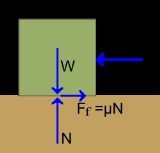xFrictionOverview

Friction is the force
Force
In physics, a force is any influence that causes an object to undergo a change in speed, a change in direction, or a change in shape. In other words, a force is that which can cause an object with mass to change its velocity , i.e., to accelerate, or which can cause a flexible object to deform...

resisting the relative motion of solid surfaces, fluid layers, and/or material elements sliding against each other. There are several types of friction:
• Dry friction resists relative lateral motion of two solid surface
Surface
In mathematics, specifically in topology, a surface is a two-dimensional topological manifold. The most familiar examples are those that arise as the boundaries of solid objects in ordinary three-dimensional Euclidean space R3 — for example, the surface of a ball...

s in contact. Dry friction is subdivided into static friction between non-moving surfaces, and kinetic friction between moving surfaces.

• Fluid friction describes the friction between layers within a viscous fluid that are moving relative to each other.

• Lubricated friction is a case of fluid friction where a fluid separates two solid surfaces.

• Skin friction is a component of drag
Drag (physics)
In fluid dynamics, drag refers to forces which act on a solid object in the direction of the relative fluid flow velocity...

, the force resisting the motion of a solid body through a fluid.

• Internal friction is the force resisting motion between the elements making up a solid material while it undergoes deformation
Deformation (mechanics)
Deformation in continuum mechanics is the transformation of a body from a reference configuration to a current configuration. A configuration is a set containing the positions of all particles of the body...

.

When surfaces in contact move relative to each other, the friction between the two surfaces converts kinetic energy
Kinetic energy
The kinetic energy of an object is the energy which it possesses due to its motion.It is defined as the work needed to accelerate a body of a given mass from rest to its stated velocity. Having gained this energy during its acceleration, the body maintains this kinetic energy unless its speed changes...

into heat
Heat
In physics and thermodynamics, heat is energy transferred from one body, region, or thermodynamic system to another due to thermal contact or thermal radiation when the systems are at different temperatures. It is often described as one of the fundamental processes of energy transfer between...

.What are some pictures that can see friction?Recall friction as a force that oppose the relative motion of the surface in contact and of the bodies moving through fluids , and describe how it occ...Factors affecting a moving object on the smooth and rough surfacesDiscussionsIf an applied force does not cause an object to move, then the type of friction acting between the surfaces is ?Friction